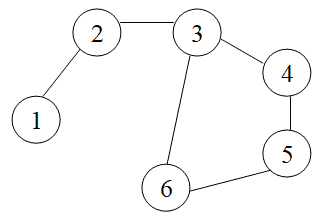## 1. Overview

In this tutorial, we’re going to learn to detect cycles in an undirected graph using Depth-First Search (DFS).

## 2. What Is a Graph?

A graph is a data structure that comprises a restricted set of vertices (or nodes) and a set of edges that connect these vertices.

We can define a graph, with a set of vertices, and a set of edges. Every edge connects two vertices, and we can show it as, whereandare connected vertices.

For example, if there is an edge between two verticesand, then we call them associated. We can then also call these two as adjacent (neighbor) vertices.

Mathematically, we can show a graph (vertices,edges) as:We can categorize graphs into two groups:

First, if edges can only be traversed in one direction, we call the graph directed.

For example, if a directed edge connects vertex 1 and 2, we can traverse from vertex 1 to vertex 2, but the opposite direction (from 2 to 1) is not allowed. So, we can say thatis not equal to.

But, if the edges are bidirectional, we call the graph undirected.

For example, if an undirected edge connects vertex 1 and 2, we can traverse from vertex 1 to vertex 2 and from 2 to 1. We can then say thatis equal to.

## 3. What Is a Cycle?

In graph theory, a path that starts from a given vertex and ends at the same vertex is called a cycle.

Cycle detection is a major area of research in computer science. The complexity of detecting a cycle in an undirected graph is.

In the example below, we can see that nodes 3-4-5-6-3 result in a cycle:## 4. Cycle Detection

Next, then, let’s learn how to detect cycles in an undirected graph. Specifically, let’s use DFS to do it.

As a quick reminder, DFS places vertices into a stack. We start with some vertexand push it onto the stack. Then:

1. Ifhas unvisited neighbors, we push one of those neighbors,, onto the stack and repeat this step (nowis)
2. Oncehas no unvisited neighbors, we pop it off of the stack and return to step 1

Now, to detect a cycle, we can adjust DFS’s logic a bit: Ifhas a visited neighborthat:

• is equal to, or
• is not equal to‘s parent

then we’ve got a cycle.

Putting this into pseudocode, we get:And now we can use it to detect cycles in undirected graphs by calling.

## 5. Conclusion

In this quick tutorial, we explored how to detect cycles in undirected graphs – basing our algorithm on Depth-First Search.